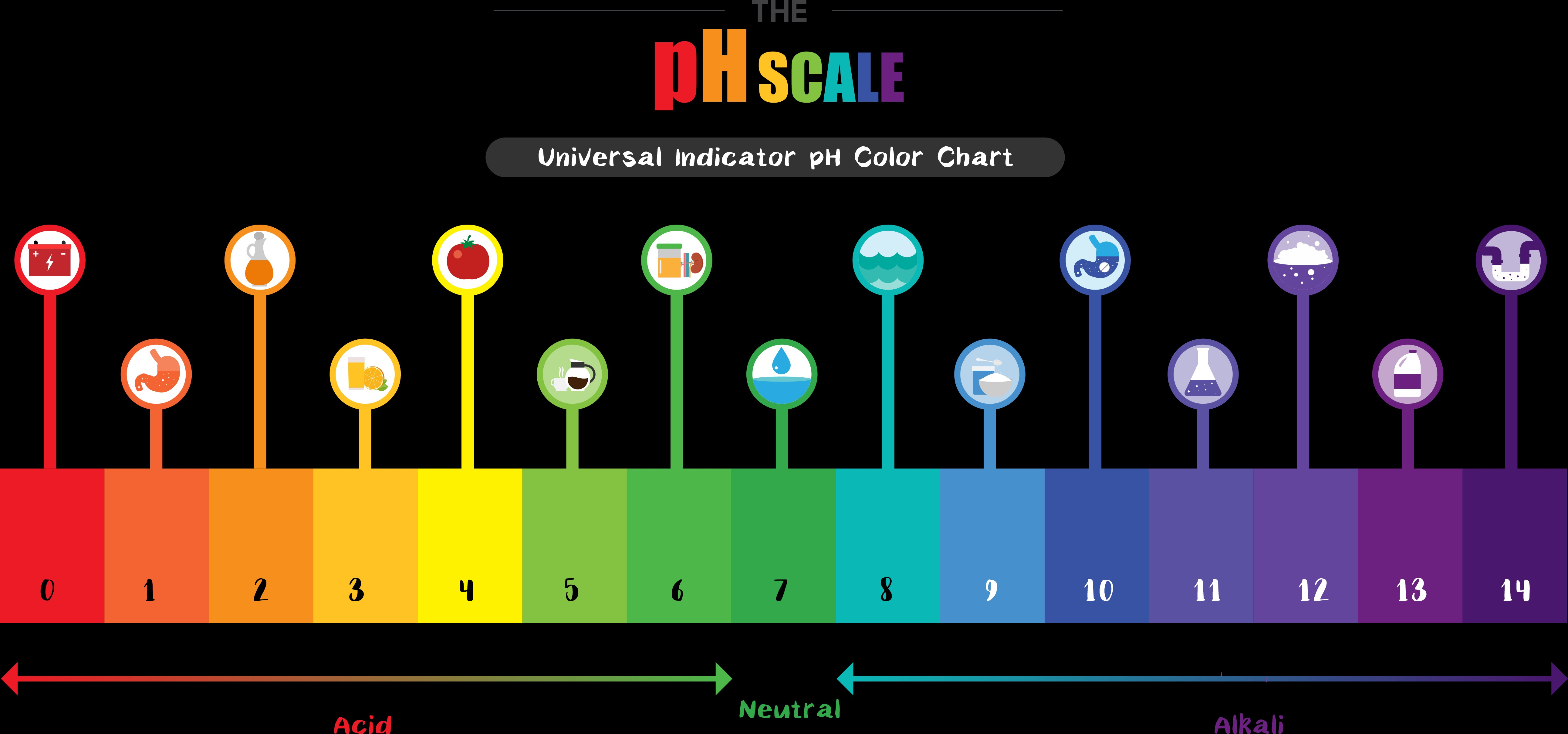Many people are aware that pH has something to do with acids or bases and that it is important for things like water quality, food-making, aquatic life and plants, but not many really understand what it means.

In simplest terms, a pH value indicates how acidic or basic water is. A pH of 7 indicates a neutral solution, a pH greater than 7 indicates a basic solution, and a pH of less than 7 indicates an acidic solution. The farther a pH value is from 7, the more strongly acidic or basic it is. For example, water with a pH of 6 is mildly acidic, and water with a pH of 3 is highly acidic.If you are satisfied with this answer, you can stop reading here. But if you want to know what pH really means, read on…

So what does pH really mean?

The ‘H’ in pH is the elemental symbol for hydrogen. The ‘p’ can refer to different things in different languages, but the ‘pH’ is most commonly said to mean ‘power of hydrogen’. So what does this mean?

The hydrogen referred to in the pH symbol is actually the hydrogen ion, which is written as H+. A hydrogen atom has one proton (which has a positive charge) and one electron (which has a negative charge). The hydrogen ion is a hydrogen atom that has given up its electron. Because there is no longer a negative charge from the electron to balance the positive charge from the proton, H+ has a net positive charge (hence the ‘+’ in the symbol).

 Technical note: Sometimes the hydrogen ion is written as the hydronium ion (H3O+).

The presence of H+ is what makes water acidic. An acid is a compound that contributes H+ to water, either directly or indirectly. For example, hydrochloric acid (HCl) dissociates into the hydrogen (H+) and chloride (Cl-) ions in water, and it is the H+ that contributes to the acidity of the water. The higher the concentration of H+, the more acidic the water is.

HCl ® H+ + Cl-

The concentration of H+ can be expressed in terms of moles per liter of water (one mole is 6.022×1023 objects, also known as Avogadro’s number). The value of pH reflects the concentration of H+ as follows:

(concentration of H+ in mol/L) = 10-pH

For example, at pH 6, the concentration of H+ is 10-6 or 0.000001 mol/L. At pH 3, the concentration is 10-3 or 0.001 mol/L.

 Technical note: Professional chemists may use slightly different definitions of pH that account for the non-ideal behavior of the hydrogen ion or that use different concentration units (such as moles per kg of water), but the above relationship explains the meaning of pH well enough for many people.

What about neutral and basic solutions?

Water molecules break apart into two ions, the hydrogen ion (H+) and hydroxyl ion (OH-):

H2O = H+ + OH-

This reaction is reversible, so that H+ and OH- can recombine to form water molecules. At any given time, the concentrations of H+ and OH- are very small compared to the amount of water molecules.

It happens that at pH 7, the concentrations of H+ and OH- are equal. This is why pH 7 is considered the neutral pH. Below pH 7, the concentration of H+ is greater than the concentration of OH-, making the water acidic. Above pH 7, the concentration of OH- is greater than the concentration of H+, making the water basic.

At high pH, the concentration of H+ is very small. For example, at pH 11, the concentration of H+ is 10-11 or 0.00000000001 mol/L. The concentration of OH-, however, is correspondingly higher. The concentration of OH- can be expressed as follows:

(concentration of OH- in mol/L) = 10pH-14

At pH 11, the concentration of OH- is 1011-14 (10-3) or 0.001 mol/L, which is the same as the concentration of H+ at pH 3.

The pH scale: the power of hydrogen

The pH scale is a logarithmic scale where a difference of one pH unit represents a power of ten difference in the concentration of H+. For example, the concentration of H+ at pH 5 is ten times higher than at pH 6, 100 times higher than at pH 7, and 1000 times higher than at pH 8. Because each pH unit represents a power of ten difference in the H+ concentration, the pH value represents the “power of hydrogen”. In fact, pH is most often defined as the negative logarithm of the H+ concentration:

pH = -log10 [H+ concentration]

The same general pattern applies to OH-. The concentration of OH- at pH 8 is ten times higher than at pH 7, 100 times higher than at pH 6, and so on.

 pH H+ concentration, mol/L OH- concentration, mol/L 0 1 1×10-14 1 0.1 1×10-13 2 0.01 1×10-12 3 0.001 1×10-11 4 0.0001 1×10-10 5 0.00001 1×10-9 6 0.000001 1×10-8 7 1×10-7 1×10-7 8 1×10-8 0.000001 9 1×10-9 0.00001 10 1×10-10 0.0001 11 1×10-11 0.001 12 1×10-12 0.01 13 1×10-13 0.1 14 1×10-14 1

A common misconception is that the pH scale begins at zero and ends at 14. Actually, negative pH values and pH values greater than 14 are possible. For example, the concentrated acids common in laboratories and industry have negative pH values. Even in the environment, pH values as low as -3.6 have been observed in extreme situations.

References

 Langmuir, D. (1997). Aqueous environmental geochemistry. Upper Saddle River, N.J: Prentice Hall.

 Nordstrom, D. K., Alpers, C. N., Ptacek, C. J., and Blowes, D. W. (2000) Negative pH and Extremely Acidic Mine Waters from Iron Mountain, California. USGS Staff -- Published Research. 479.